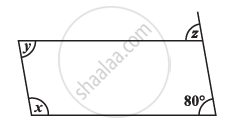Share

# Consider the Given Parallelograms. Find the Values of the Unknowns X, Y, Z. - 4 - CBSE Class 8 - Mathematics

ConceptKinds of Quadrilaterals Concept of Parallelogram

#### Question

Consider the given parallelograms. Find the values of the unknowns x, y, z.#### Solution

z = 80° (Corresponding angles)

y = 80° (Opposite angles are equal)

x+ y = 180° (Adjacent angles are supplementary)

x = 180° − 80° = 100°

Is there an error in this question or solution?

#### APPEARS IN

NCERT Solution for Mathematics Textbook for Class 8 (2018 to Current)